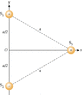# Coulomb law point charges

• tuggler

## Homework Statement

The sides of the triangular web have a length of a = 0.74 m, as depicted in the figure. Two of the spiders (S1 and S3) have +6.6 µC charge, while the other (S2) has −6.6 µC charge.

a.) What are the magnitude and direction of the net force on the third spider (S3)?

I attached the image below because I don't know how to link it through here.

## Homework Equations

$$|\boldsymbol{F}|=k_e{|q_1q_2|\over r^2}$$

## The Attempt at a Solution

There is a force vector on S3 pointing away from the positive charge of S1 and there is another force vector pointing towards charge S2. I applied Coulomb's law for the force vector on S1 to S3 and I got the distance r = 0.74 but I can't find the angle. Will the angle just be $$\tan^{-1}(.37/.64)$$ so I will get:

$$|\boldsymbol{F}|=k_e{|(6.6\times 10^{-6})^2 |\over (0.74)^2}(\cos\theta I - \sin\theta j)$$

#### Attachments

•18-p-047.gif
4.2 KB · Views: 796
Last edited:

## Homework Statement

The sides of the triangular web have a length of a = 0.74 m, as depicted in the figure. Two of the spiders (S1 and S3) have +6.6 µC charge, while the other (S2) has −6.6 µC charge.

a.) What are the magnitude and direction of the net force on the third spider (S3)?

I attached the image below because I don't know how to link it through here.

## Homework Equations

$$|\boldsymbol{F}|=k_e{|q_1q_2|\over r^2}$$

## The Attempt at a Solution

There is a force vector on S3 pointing away from the positive charge of S1 and there is another force vector pointing towards charge S2. I applied Coulomb's law for the force vector on S1 to S3 and I got the distance r = 0.74 but I can't find the angle. Will the angle just be $$\tan^{-1}(.37/.64)$$ so I will get:

$$|\boldsymbol{F}|=k_e{|(6.6\times 10^{-6})^2 |\over (0.74)^2}(\cos\theta I - \sin\theta j)$$

No, what angle you speak about? Angle with respect to what?

Draw the force vectors acting S3, and draw their vector sum. The magnitude of the Coulomb forces both from S1 and S2 are the same, only the directions differ. What can be the direction of the resultant force?

ehild